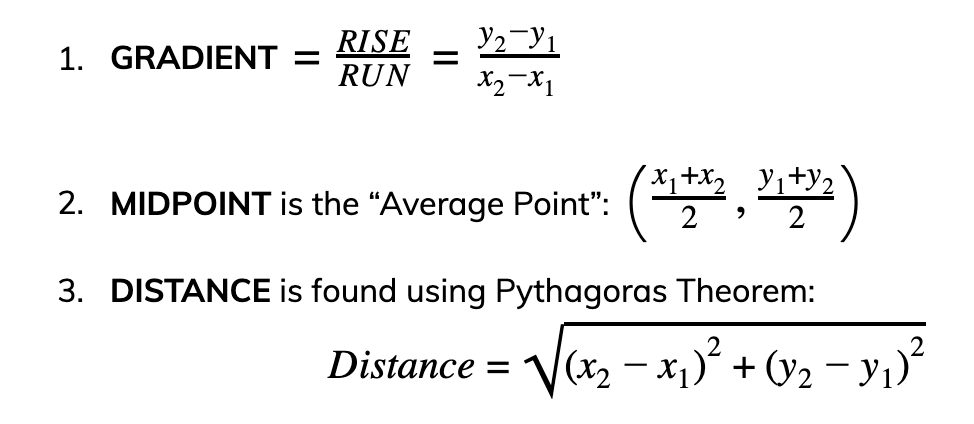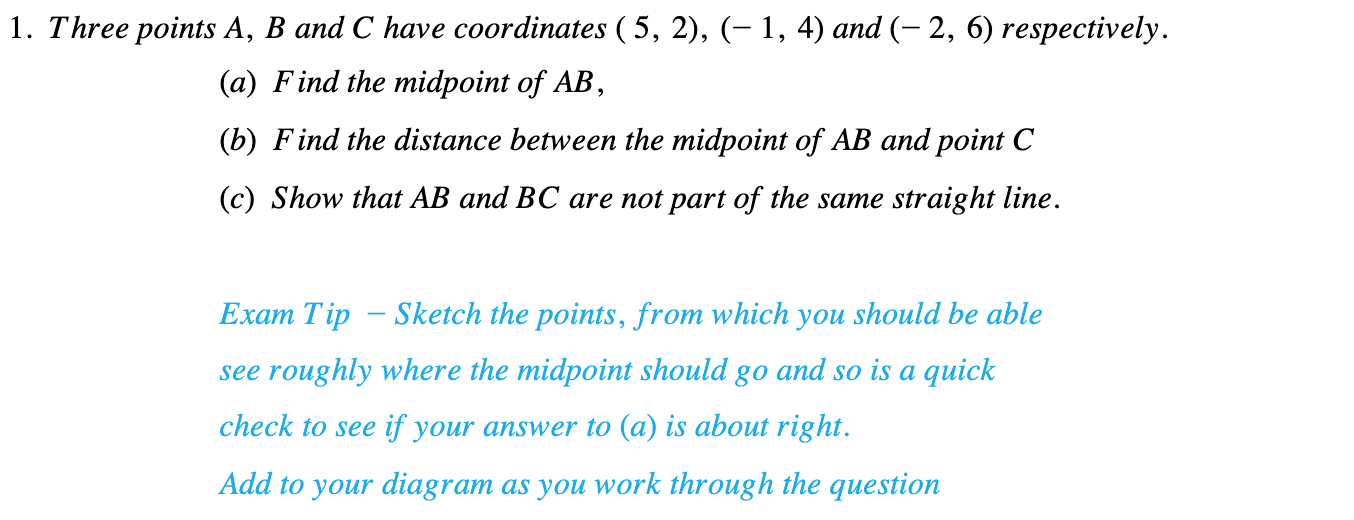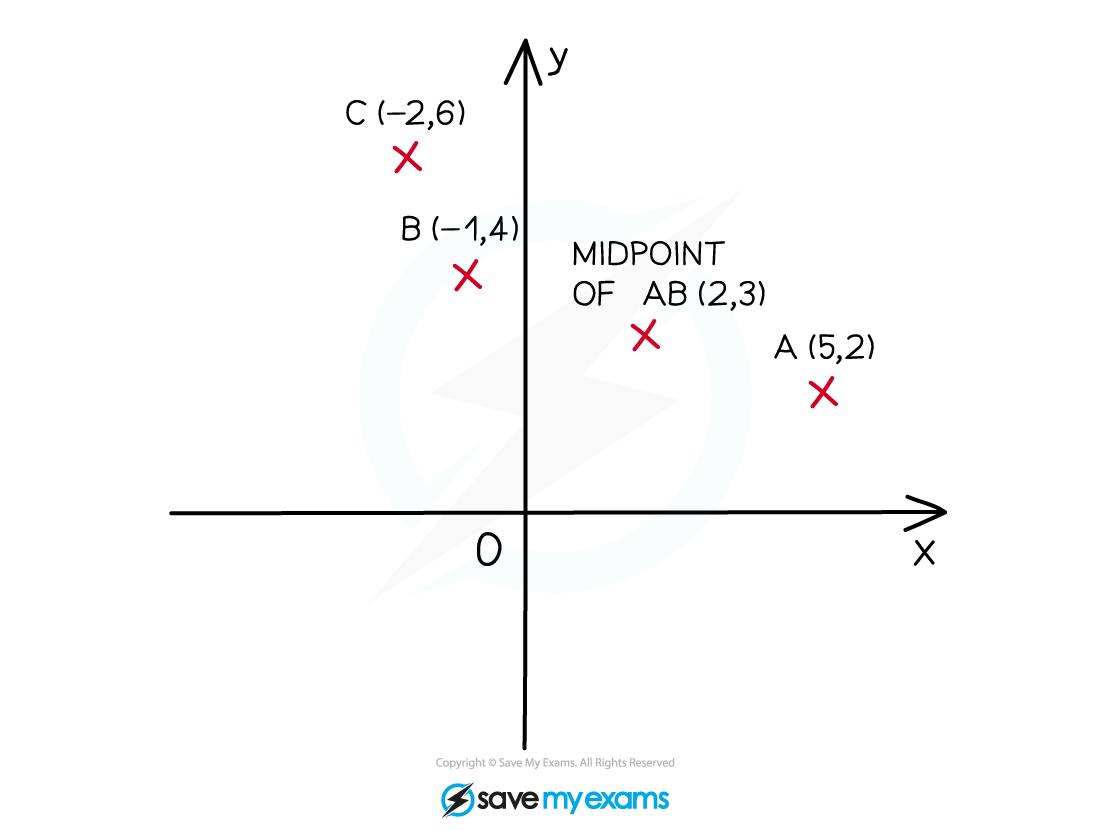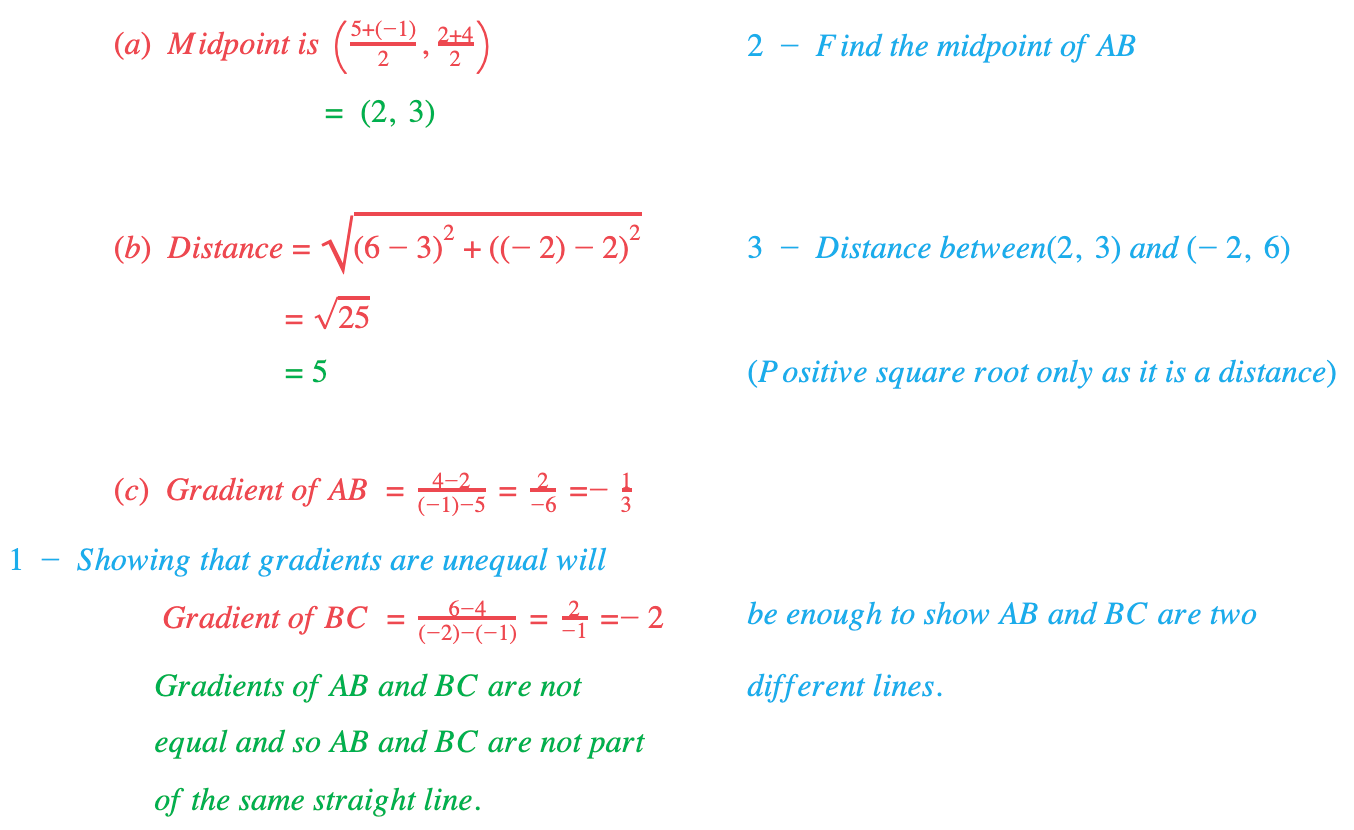# Edexcel IGCSE Maths 复习笔记 3.5.1 Coordinates

Edexcel IGCSE Maths 复习笔记 3.5.1 Coordinates

#### What are coordinates?

• When we want to plot a point on a graph we need to know where to put it
• If the horizontal axis is labelled x and the vertical axis is labelled y, then the x and y coordinates are how far we go along the x and y axes to plot the point

#### What can we do with coordinates?

• If we have two points with coordinates (x1, y1) and (x2, y2) then we should be able to find
• the gradient of the line through them
• the midpoint of the two points
• the distance between the two points

• Don’t get fazed by the horrid notation (x1, y1) – this is just “point 1” and the other is “point 2” so we put the little numbers (subscripts) in so that we know which coordinate we are referring to. In questions there’ll be lots of nice numbersHere’s how we do each of those:• You should also know that three points A, B and C lie on the same STRAIGHT LINE if AB and AC (or BC) have the same gradient

#### Exam Tip

If in doubt, SKETCH IT!

A quick, reasonably accurate etch can make things a lot clearer.

All of the above can also be applied to 3D coordinates in the form (x, y, z), on a 3D axis.

#### Worked Example#### Worked Example